# Getting started with wrGraph

## Introduction

This package contains a collection of various plotting tools, mostly as an extension of packages wrMisc and wrProteo.

This package is available from CRAN, you might also have to install wrMisc, too. If not yet installed, the lastest versions of this package can be installed like this :

install.packages("wrGraph")

You cat start the vignette for this package by typing :

# access vignette :
browseVignettes("wrGraph")    #  ... and the select the html output

To get started, we need to load the packages wrMisc and wrGraph (this package).

library("wrMisc")
library("wrGraph")
# This is version no:
packageVersion("wrGraph")
#>  '1.2.5'

## Prepare Layout for Accomodating Multiple Smaller Plots

The function partitionPlot() prepares a matrix to serve as grid for segmenting the current device (ie the available plotting region). It aims to optimize the layout based on a given number of plots to accommodate. The user may choose if the layout should rather be adopted to landscape (default) or portrait geometry.

This might be useful in particular when during an analysis-pipeline it’s not known/clear in advance how many plots might be needed in a single figure space.

## as the last column of the iris-data is not numeric we choose -1
(part <- partitionPlot(ncol(iris)-1))
#>      [,1] [,2]
#> [1,]    1    2
#> [2,]    3    4
layout(part)
for(i in colnames(iris)[-5]) hist(iris[,i], main=i)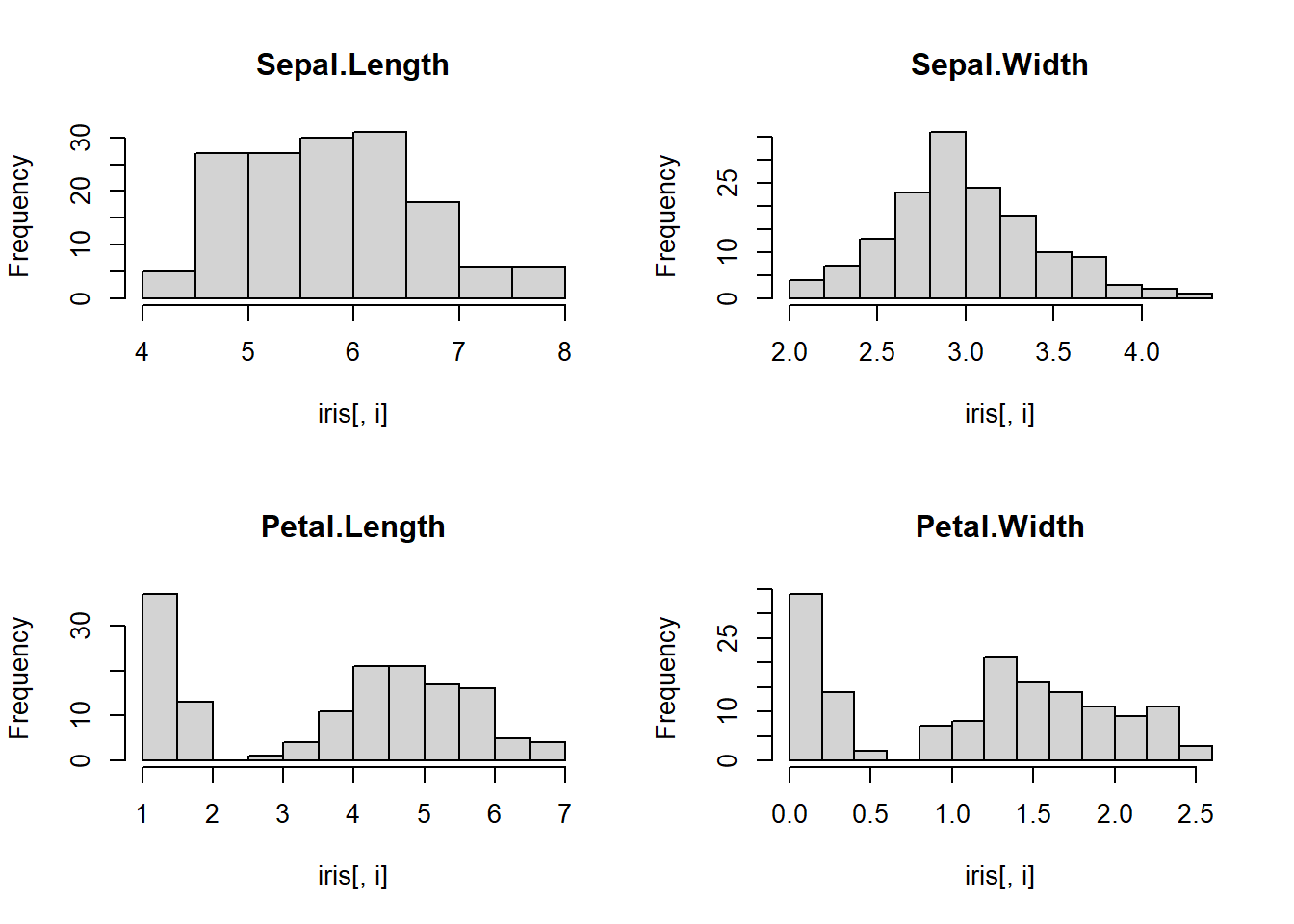## Optimal Legend Location

The function checkForLegLoc() allows to check which corner of a given graph is less crowded for placing a legend there. Basic legends can be added directly, or one can simply recuperate the location information.

dat1 <- matrix(c(1:5,1,1:5,5), ncol=2)
grp <- c("abc","efghijk")
(legLoc <- checkForLegLoc(dat1, grp))
#> $showL #>  TRUE #> #>$loc
#>  "bottomright"
#>
#> $nConflicts #> topleft topright bottomright bottomleft #> 1 1 0 1 # now with more graphical parameters (using just the best location information) plot(dat1, cex=2.5, col=rep(2:3,3),pch=rep(2:3,3)) legLoc <- checkForLegLoc(dat1, grp, showLegend=FALSE) legend(legLoc$loc, legend=grp, text.col=2:3, pch=rep(2:3), cex=0.8)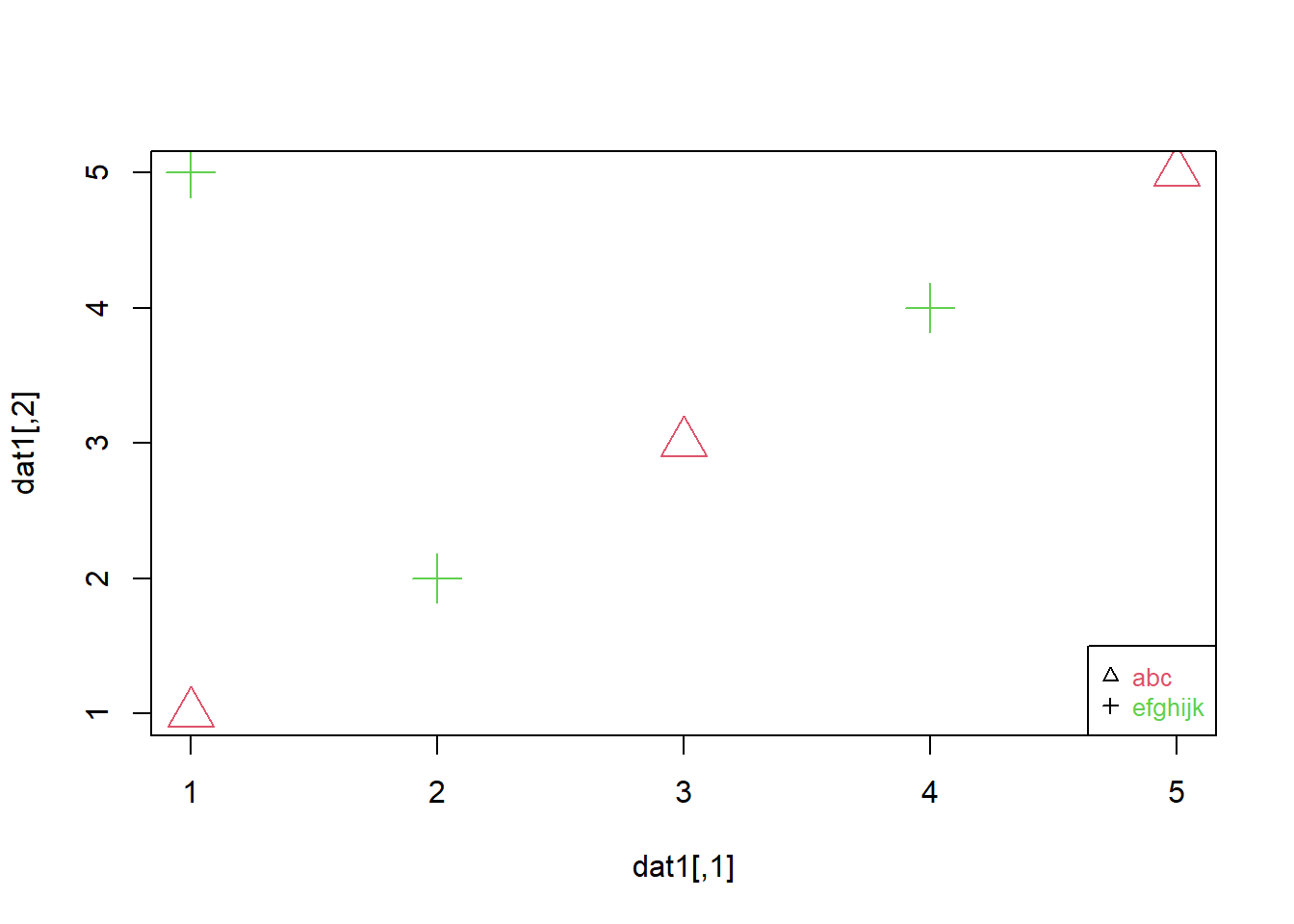## One More Histogram Function …

Histograms are a very versatile tool for rapidly gaining insights in the distribution of data. This package presents a histogram function allowing to conveniently work with log2-data and (if desired) display numbers calculated back to linear values on the x-axis.

Default settings aim to give rather a quick overview, for “high resolution” representations one could set a high number of breaks or one might also consider other/alternative graphical representations. Some of the alternatives are shown later in this vignette.

set.seed(2016); dat1 <- round(c(rnorm(200,6,0.5), rlnorm(300,2,0.5), rnorm(100,17)),2)
dat1 <- dat1[which(dat1 <50 & dat1 > 0.2)]
histW(dat1, br="FD", isLog=FALSE)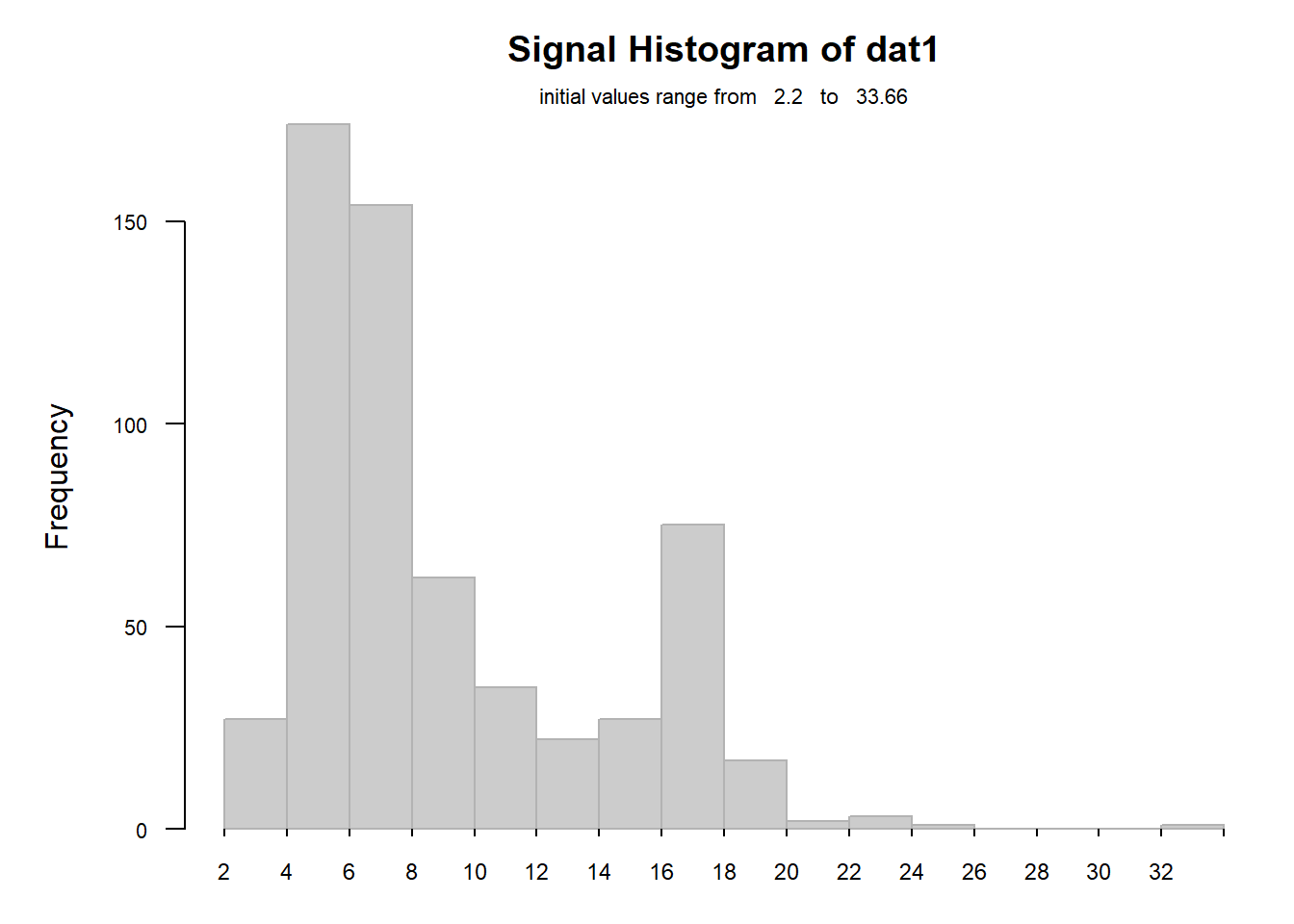One interesting feature is the fact that this fucntions can handle log-data (and display x-axis classes as linear) :

## view as log, but x-scale in linear
histW(log2(dat1), br="FD", isLog=TRUE, silent=TRUE)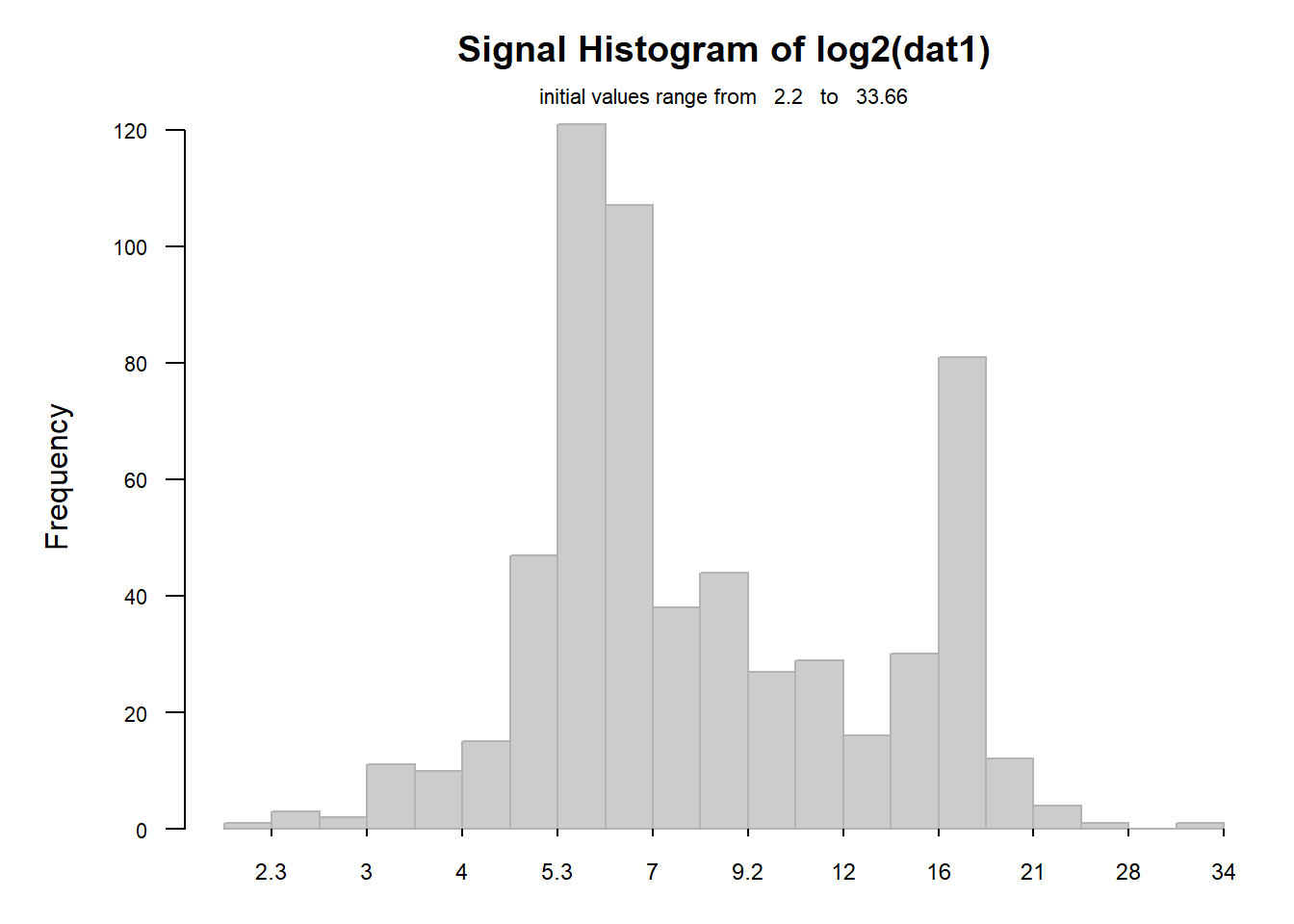Now we can combine this with the previous segmentation to accomodate 4 histograms :

## quick overview of distributions
layout(partitionPlot(4))
for(i in 1:4) histW(iris[,i], isLog=FALSE, tit=colnames(iris)[i])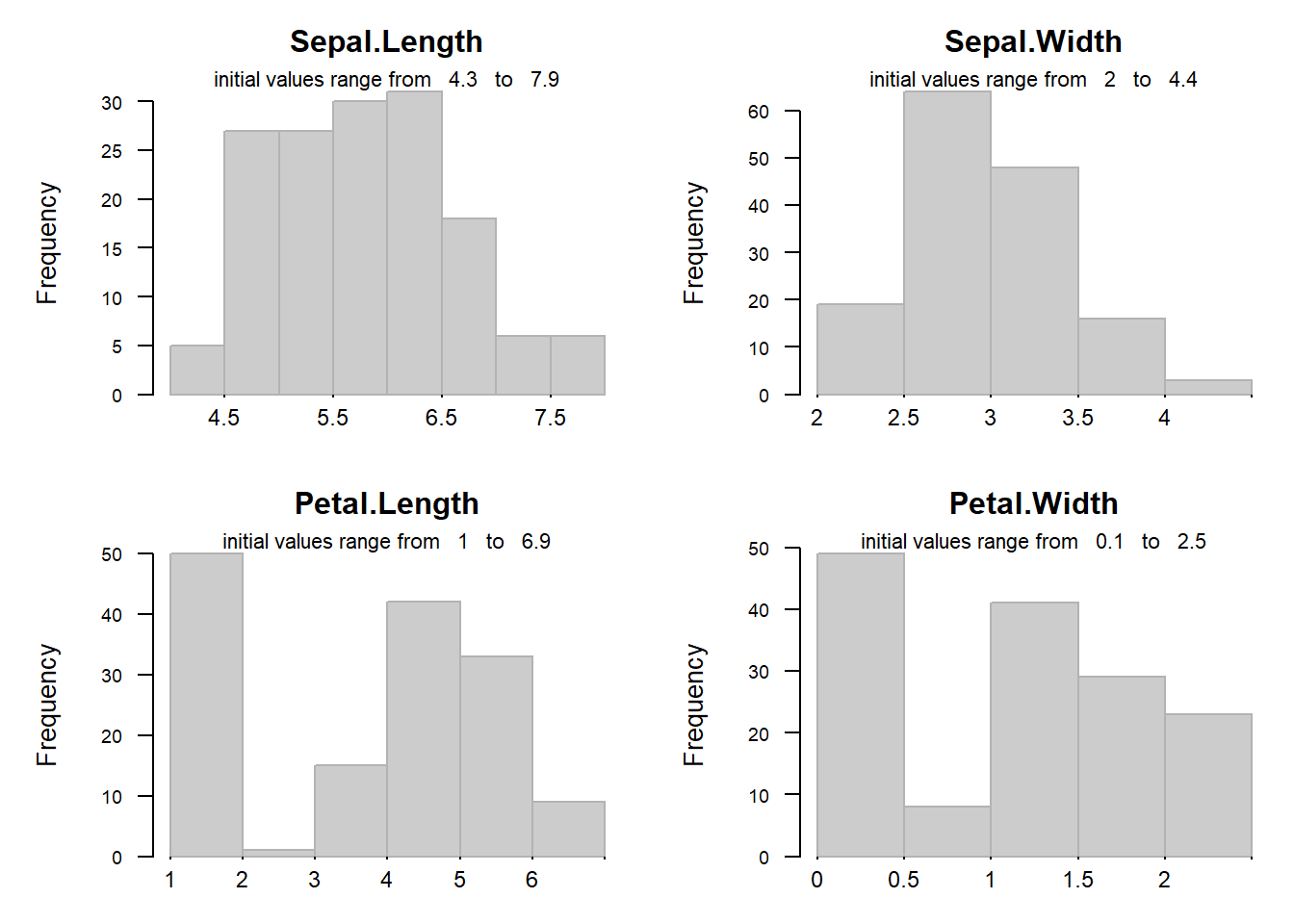### Small Histogram(s) as Legend

With some plots it may be useful to add small histograms for the x- and/or y-data.

layout(1)
plot(iris[,1:2], col=rgb(0.4,0.4,0.4,0.3), pch=16, main="Iris data")
legendHist(iris[,1], loc="br", legTit=colnames(iris), cex=0.5)
legendHist(iris[,2], loc="tl", legTit=colnames(iris), cex=0.5)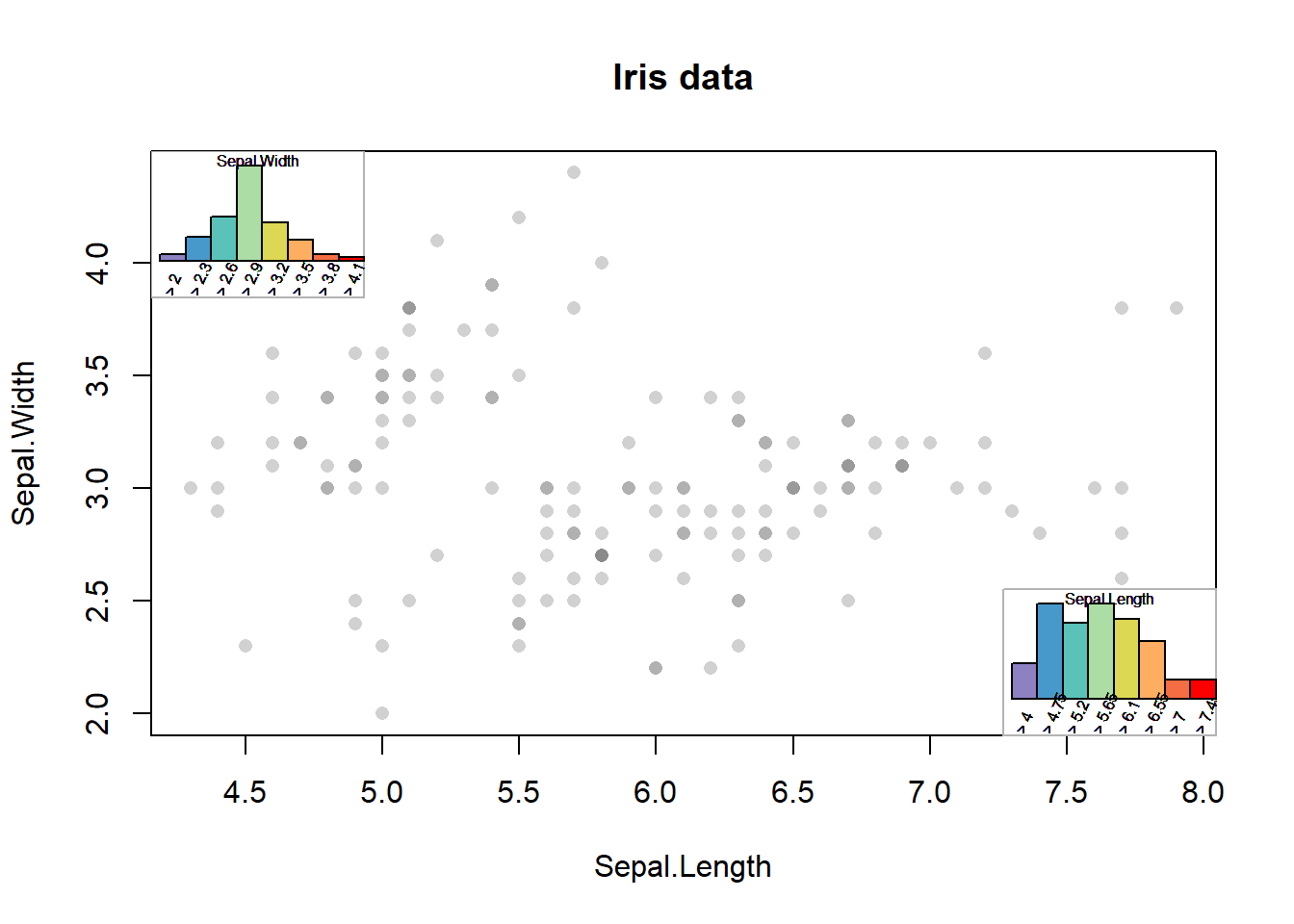## Violin Plots

Violin plots or vioplots are basically an adaptation of plotting the Kernel density estimation allowing to compare multiple data-sets. Please note, that although smoothed distributions please the human eye, some data-sets do not have such a continuous character.

Compared to the ‘original’ vioplots in R from package vioplot, the function provided here offers more flexibility for data-formats accepted (including data.frames and lists), coloring and display of n. In the case of the iris-data, there are no NAs and thus n is constant, thus the number of values (n) will be displayed only once. However, when working with data-sets containing NAs, or simply when working with lists the number of values per data-set/violin n may vary.

vioplotW(iris[,-5],tit="Iris-data")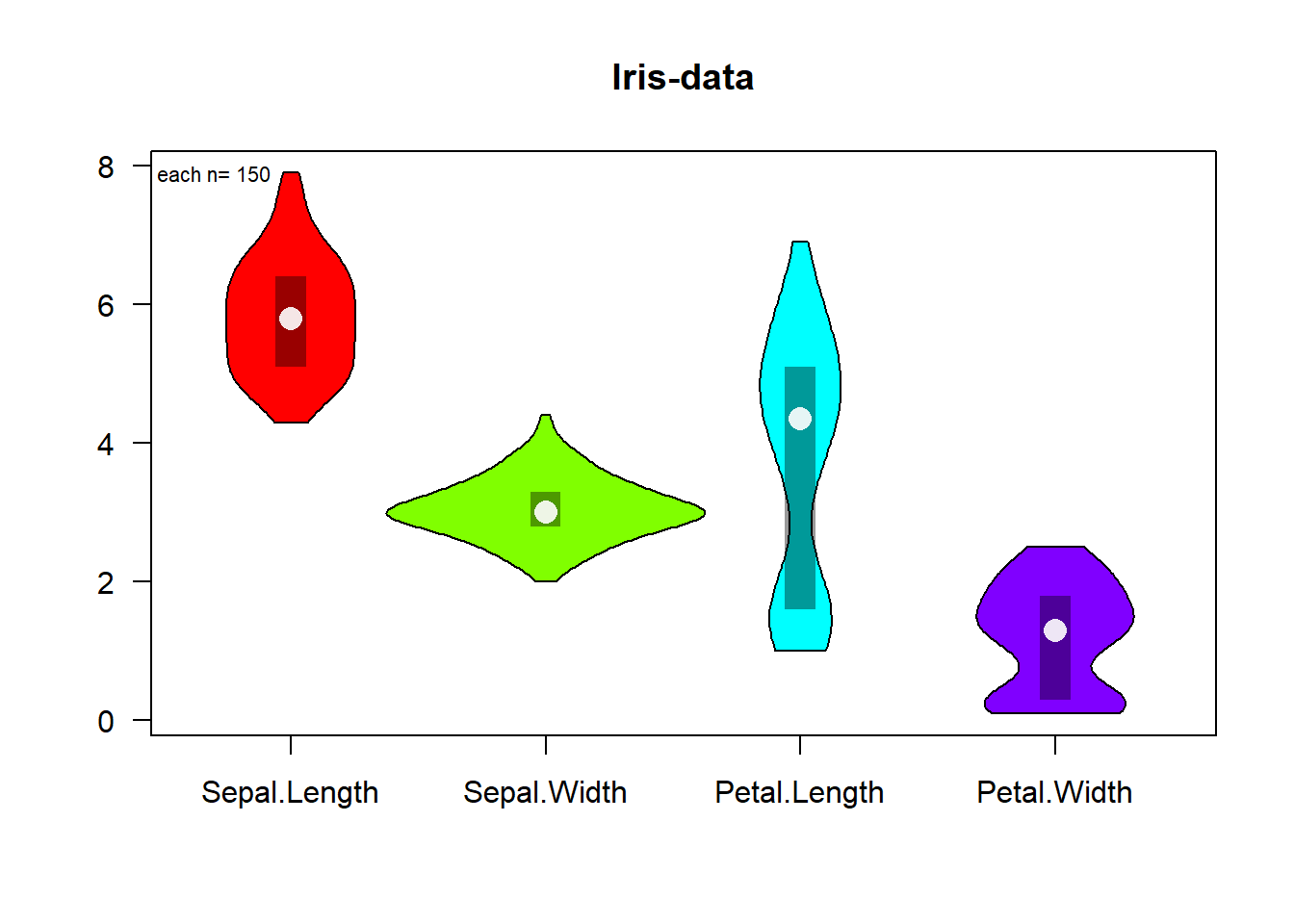## Plotting Sorted Values (‘Summed Frequency’)

This plot offers an alternative to histograms and density-plots. While histograms and density-plots are very intuitive, their interpretation may pose some difficulties due to the smoothing effect of Kernel-functions or the non-trivial choice of optimal width of bars (histogram) may influence interpretation.

As alternative, a plot is presented which basically reads like a summed frequency plot and has the main advantage, that all points of data may be easily displayed. Thus the resultant plot does not suffer from deformation due to binning or smoothing and offers maximal ‘resolution’.

cumFrqPlot(iris[,1:4])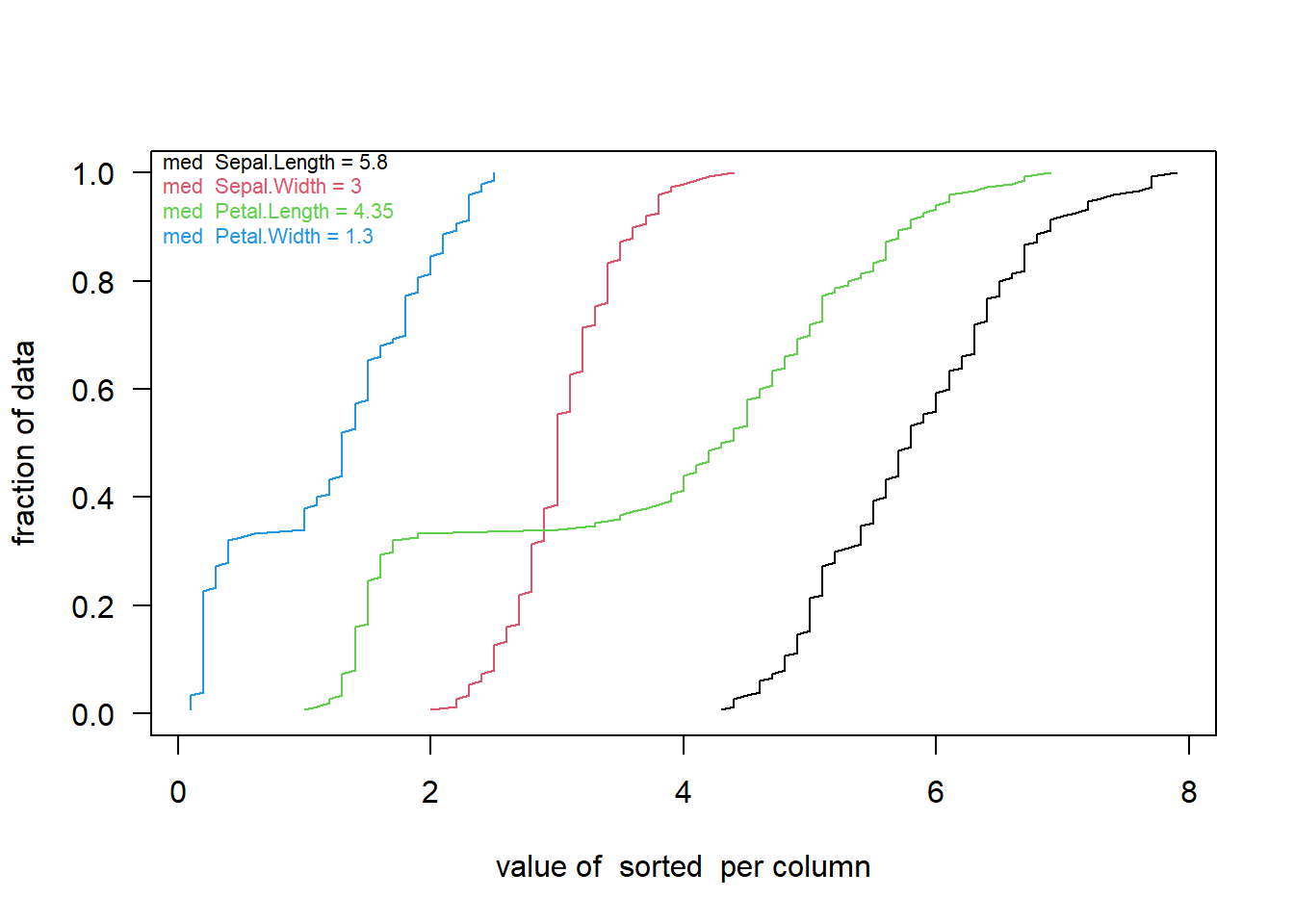For example, the iris-data are rounded. As a result in the plot above the line is not progressing smooth but with more marked character of steps of stairs. To get the same conclusion one would need to increase the number of bars in a histogram very much which would makes it in our experience more difficult to evaluate the same time the global distribution character.

At this plot you may note that the curves patal.width and petal.length look differently. On the previous vioplots you may have noticed the bimodal character of the values, again this plot may be helpful to identify distributions which are very difficult to see well using boxplots.

## Color-Code Numeric Content of Matrix (Heatmap)

To get a quick overview of the distribution of data and, in particular, of local phenomena it is useful to express numeric values as colored boxes. The function image() from the graphic-package provides basic help. Such an output can be referred to as heatmap. The function imageW() from this package offers convenient options for displaying row- and column-names.

par(mar=c(4, 5.5, 4, 1))
imageW(as.matrix(iris[1:40,1:4]), tit="Iris-data")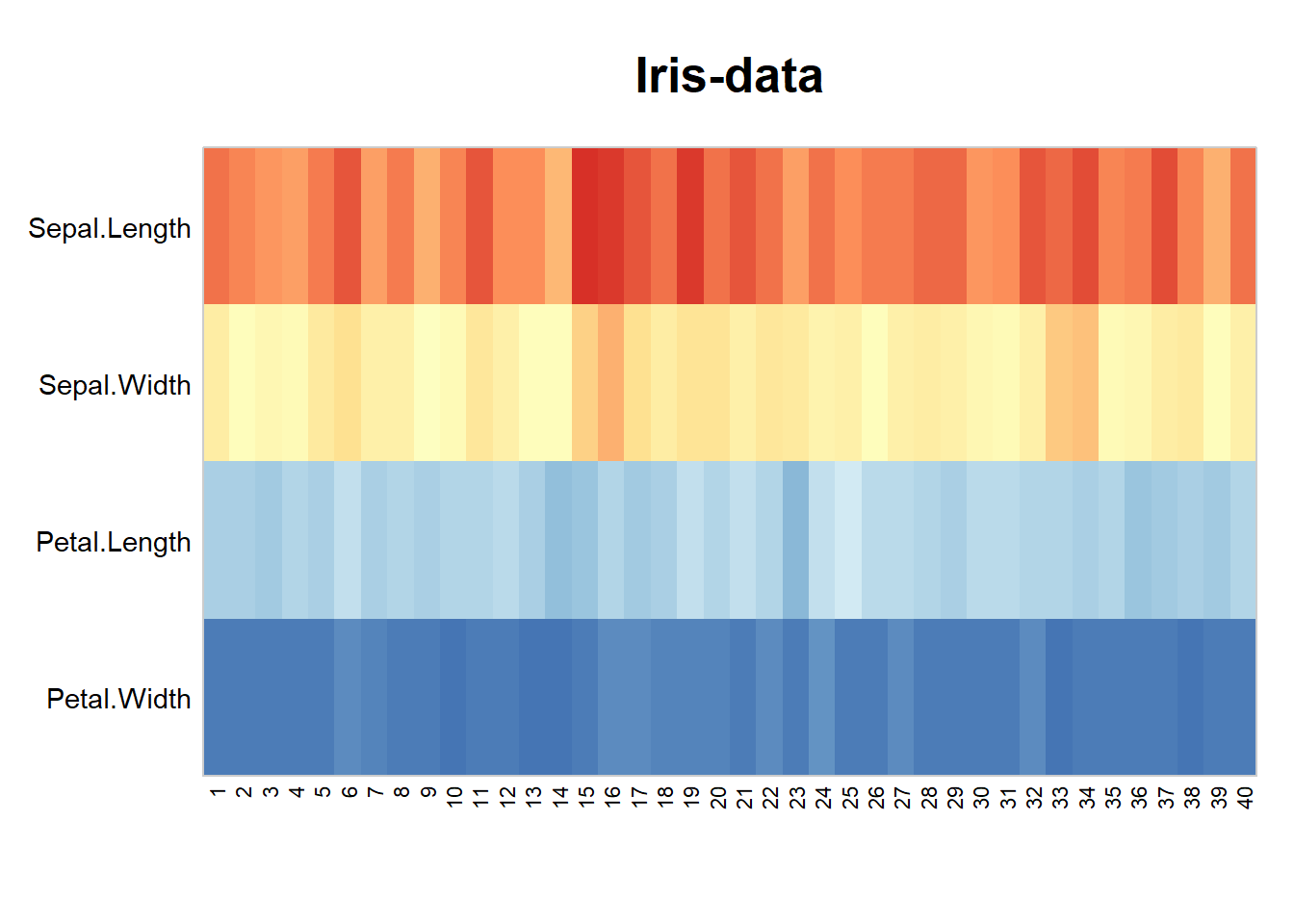Heatmaps are well known for displaying the results of hierarchical clustering, see also for example heatmap() (stats-package) or heatmap.2() from the gplots-package. In these case rows and columns of the data also get reorganized and dendrograms attached.

## Examine Counts based on Variable Threshold Levels (for ROC curves)

The next plot is dedicated to visualize counting results with moving thresholds. While a given threshold criteria moves up or down the resulting number of values passing may not necessarily follow in a linear way. This function lets you follow multiple types of samples (eg type of leave in the iris-data) in a single plot. In particular when constructing ROC curves it may also be helpful to visualize the (absolute) counting data used underneath before determining TP and FP ratios. Typically used in context of benchmark-tests in proteomics.

thr <- seq(min(iris[,1:4]), max(iris[,1:4])+0.1,length.out=100)
irisC <- sapply(thr,function(x) colSums(iris[,1:4] < x))
irisC <- cbind(thr,t(irisC))

#>            thr Sepal.Length Sepal.Width Petal.Length Petal.Width
#> [1,] 0.1000000            0           0            0           0
#> [2,] 0.1797980            0           0            0           5
#> [3,] 0.2595960            0           0            0          34
#> [4,] 0.3393939            0           0            0          41
#> [5,] 0.4191919            0           0            0          48
#> [6,] 0.4989899            0           0            0          48
staggerdCountsPlot(irisC[,], countsCol=colnames(iris)[1:4], tit="Iris-data")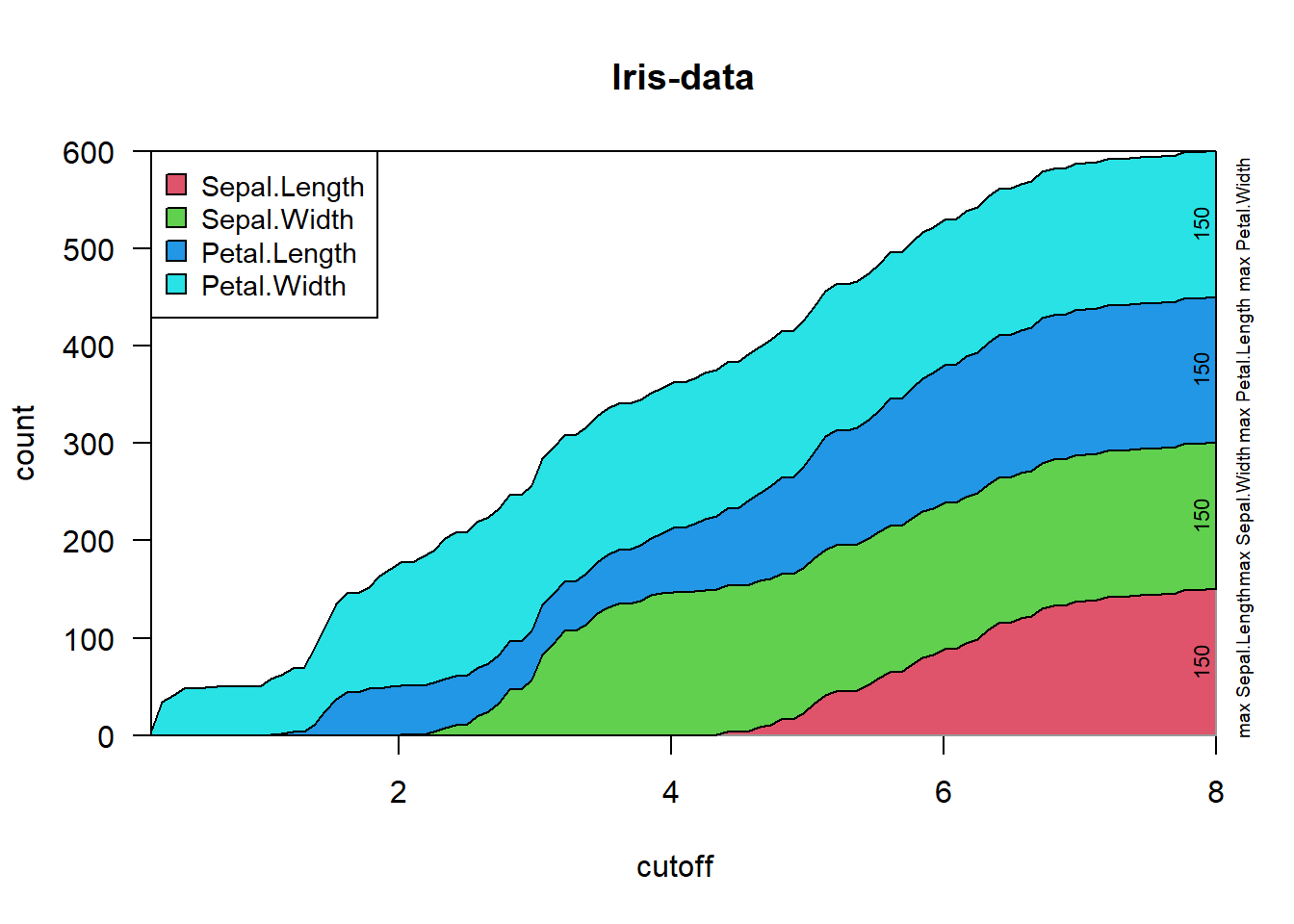staggerdCountsPlot(irisC[,], varCountNa="Sepal", tit="Iris-data")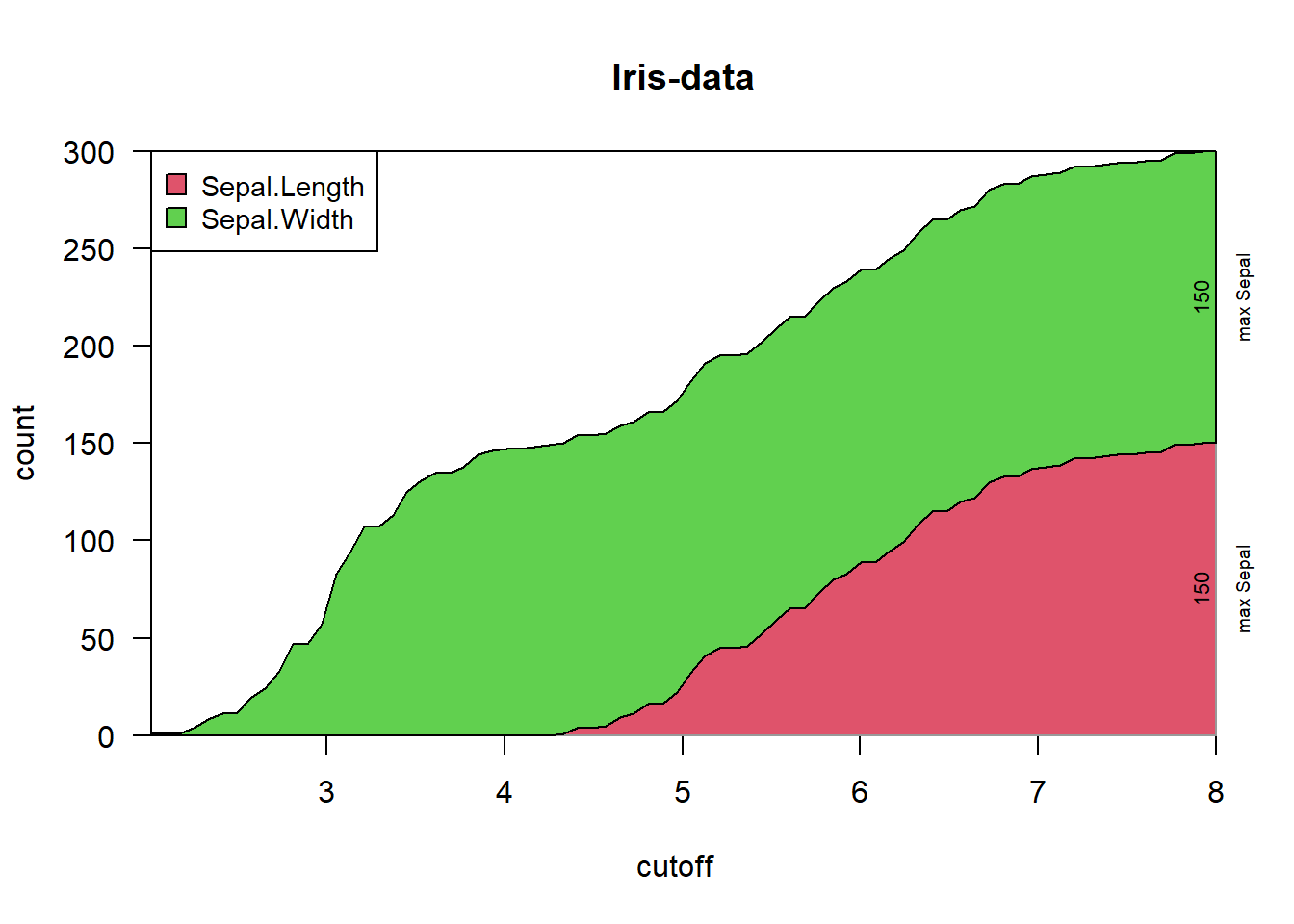staggerdCountsPlot(irisC[,], varCountNa="Sepal", tit="Iris-data (log-scale)", logScale=TRUE)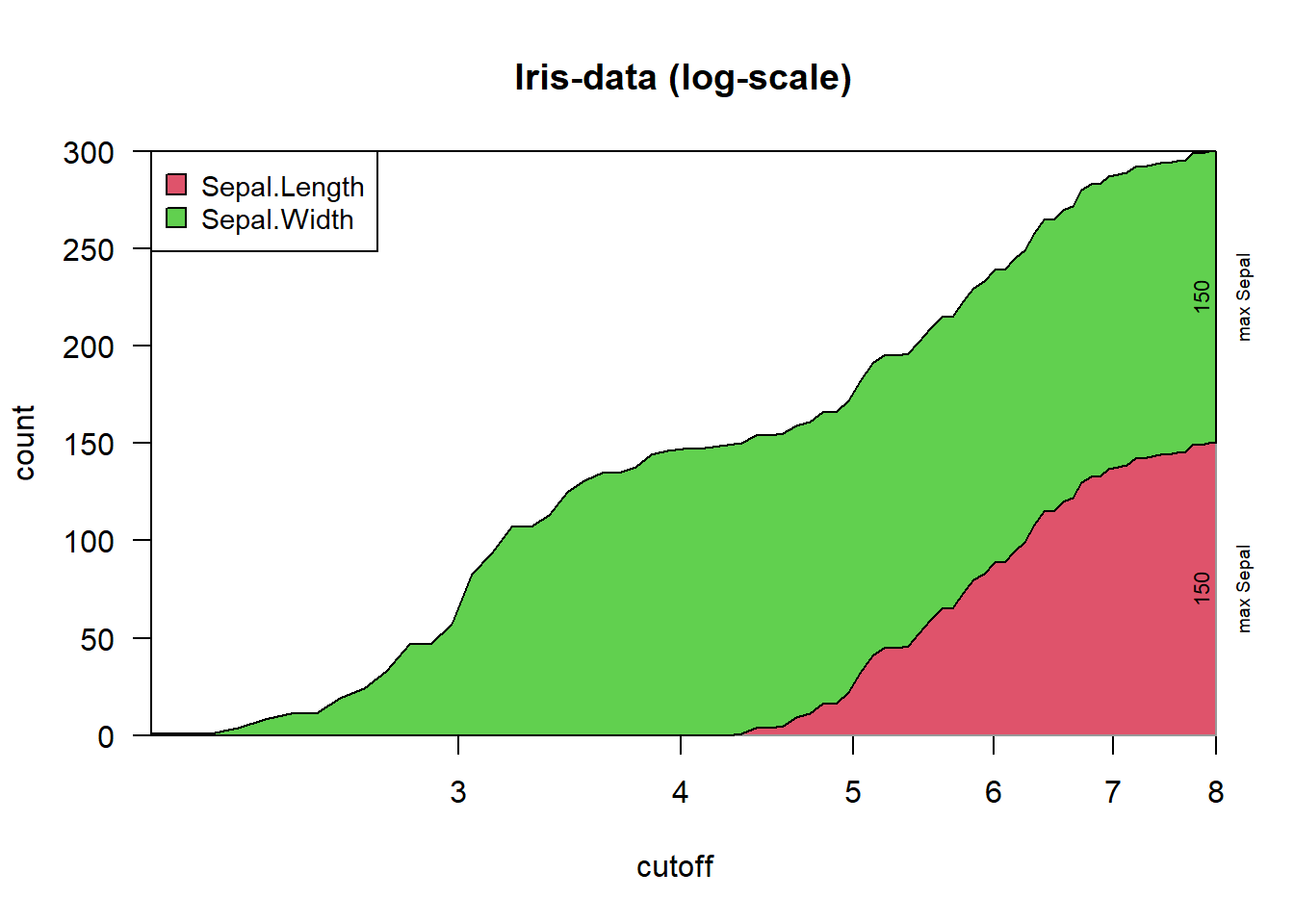## Compare Two Groups with Sub-Organisation Each

In real-world testing data have often some nested structure. For example repeated measures from a set of patients which can be organized as diseased and non-diseased. This plot allows to plot all values obtained from the each patient together, then organized by disease-groups.

For this example suppose the iris-data were organized as 10 sets of 5 measures each (of course, in this case it is a pure hypothesis). Then, we can plot while highlighting the two factors (ie species and set of measurement). Basically we need to furnish with the main data two additional factors for the groupings. Note, that the 1st factor should contain the smaller sub-groups to visually inspect if there are any batch effects. This plot is not well adopted to big data (it will get too crowded).

dat <- iris[which(iris$Species %in% c("setosa","versicolor")),] plotBy2Groups(dat$Sepal.Length, gl(2,50,labels=c("setosa","versicolor")),
gl(20,5), yLab="Sepal.Length")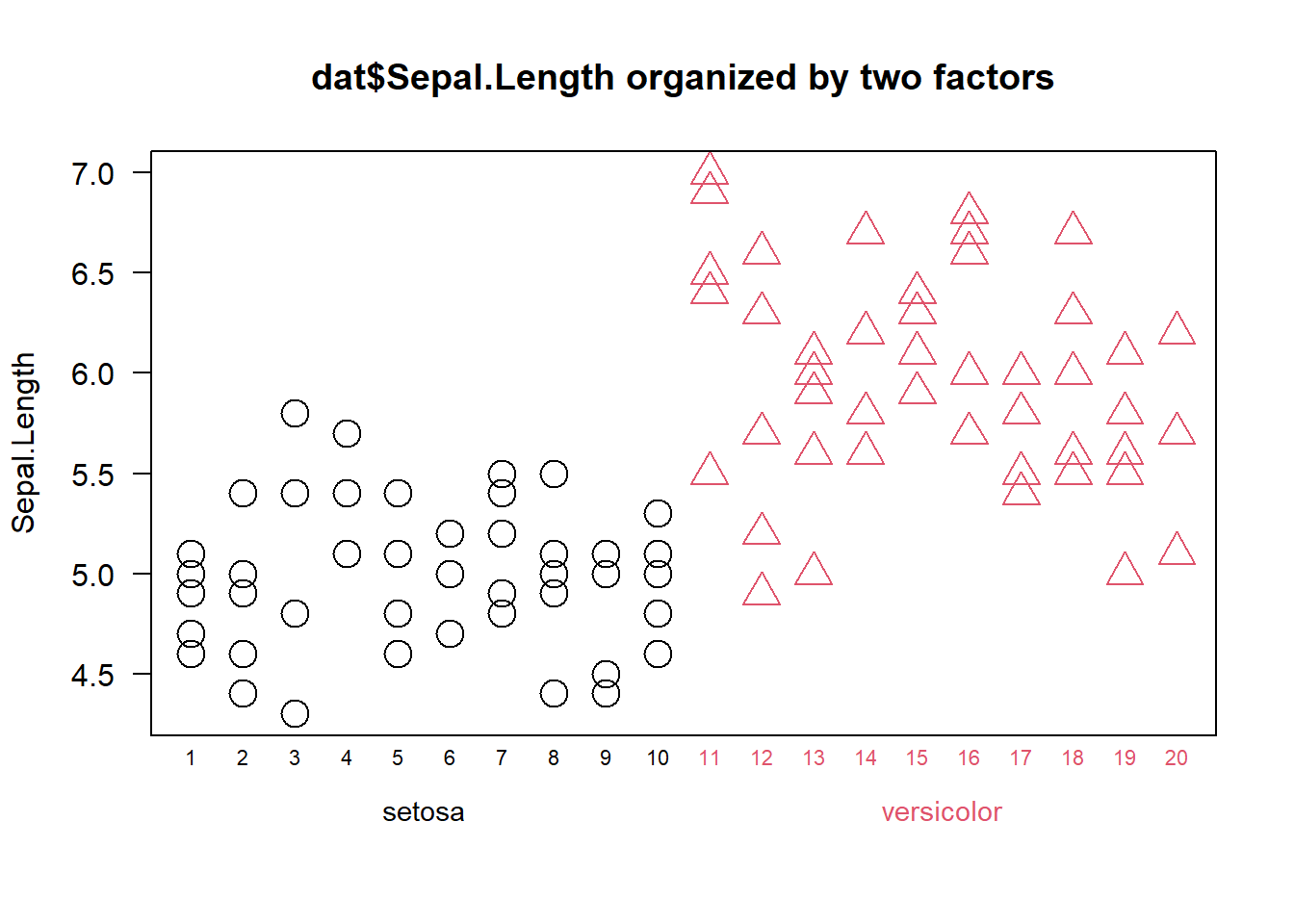## Plotting Linear Regression and Confidence Intervals

The function plotLinReg() provides help to display a series of bivariate points given in ‘dat’ (multiple data formats possible), to model a linear regression and plot the results.

plotLinReg(iris$Sepal.Length, iris$Petal.Width, tit="Iris-data")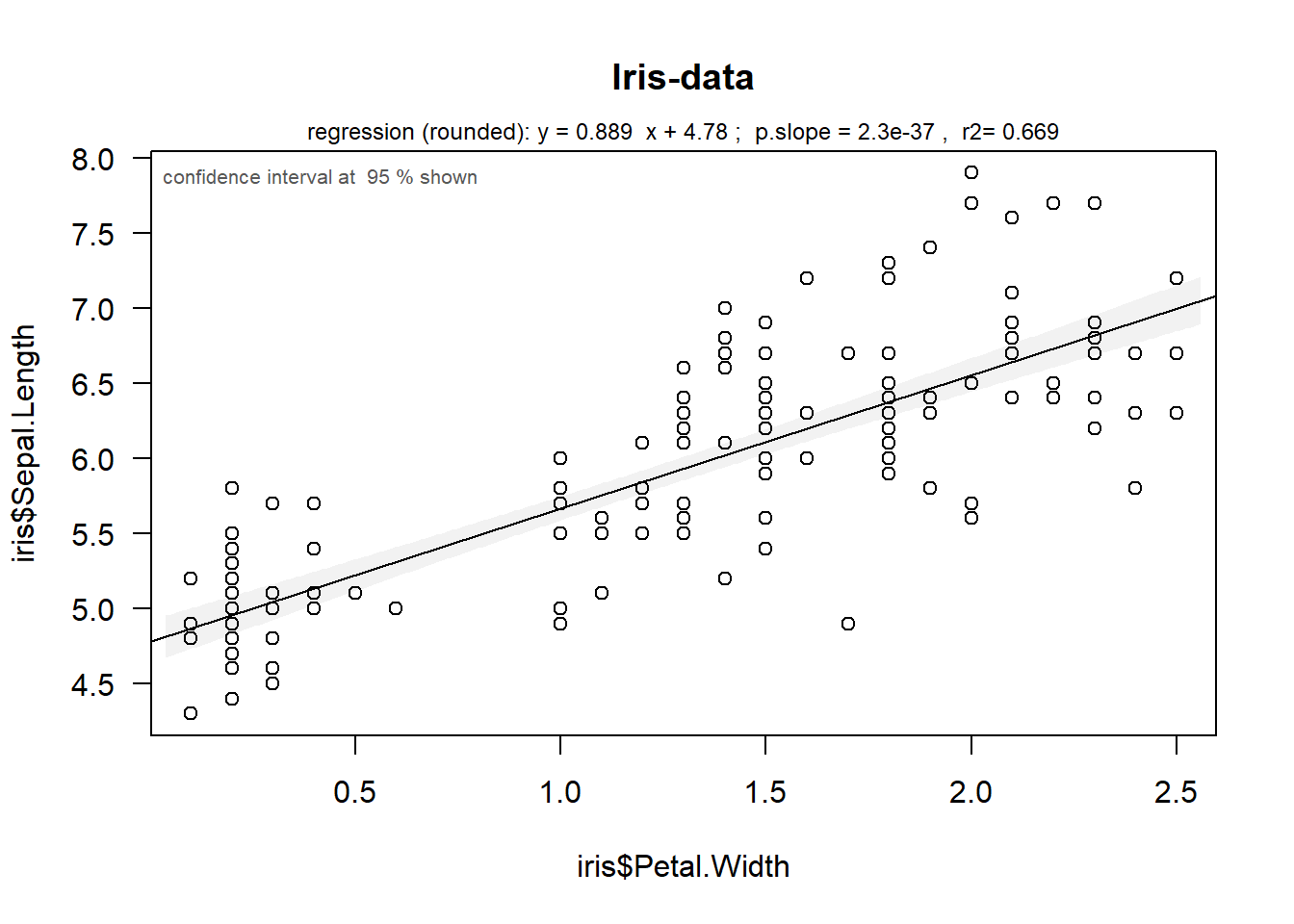## Principal Components Analysis (PCA)

Principal components analysis, PCA, is a very powerful method to investigate similarity and correlation in larger sets of data.
Please note that several implementations exist in R (eg prcomp() in the base package stats or the package FactoMineR). We’ll start by looking at the plot produced with the basic function and FactoMineR, too.

Let’s look at the similarity of the 3 iris-species from the iris data-set.

## the basic way
iris.prc <- prcomp(iris[,1:4], scale.=TRUE)
biplot(iris.prc)              # traditional plot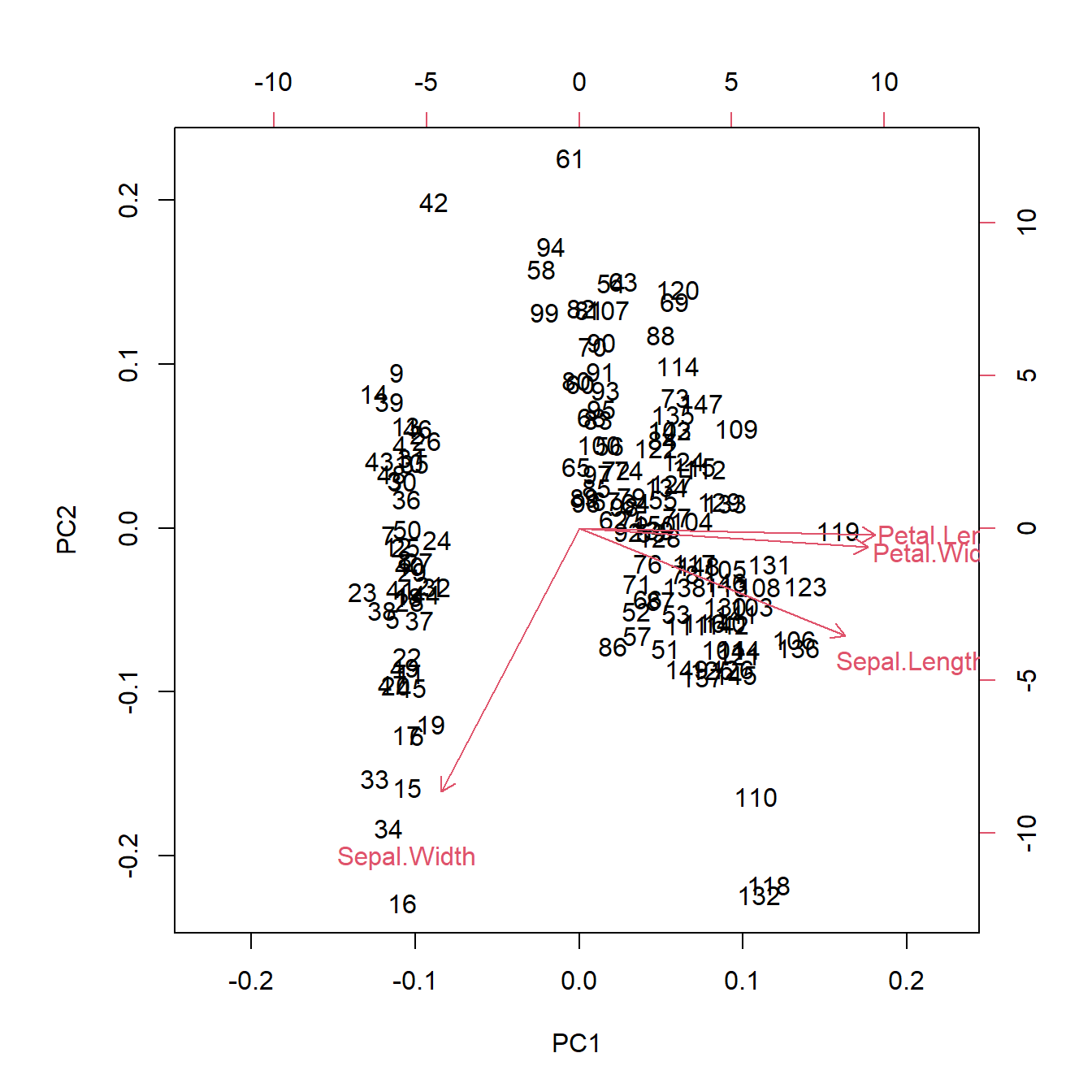## via FactoMineR
library(FactoMineR); library(dplyr); library(factoextra)
#>
#> Attaching package: 'dplyr'
#> The following objects are masked from 'package:stats':
#>
#>     filter, lag
#> The following objects are masked from 'package:base':
#>
#>     intersect, setdiff, setequal, union
iris.Fac <- PCA(iris[,1:4],scale.unit=TRUE, graph=FALSE)
fviz_pca_ind(iris.Fac, geom.ind="point", col.ind=iris$Species, palette=c(2,4,3), addEllipses=TRUE, legend.title="Groups" )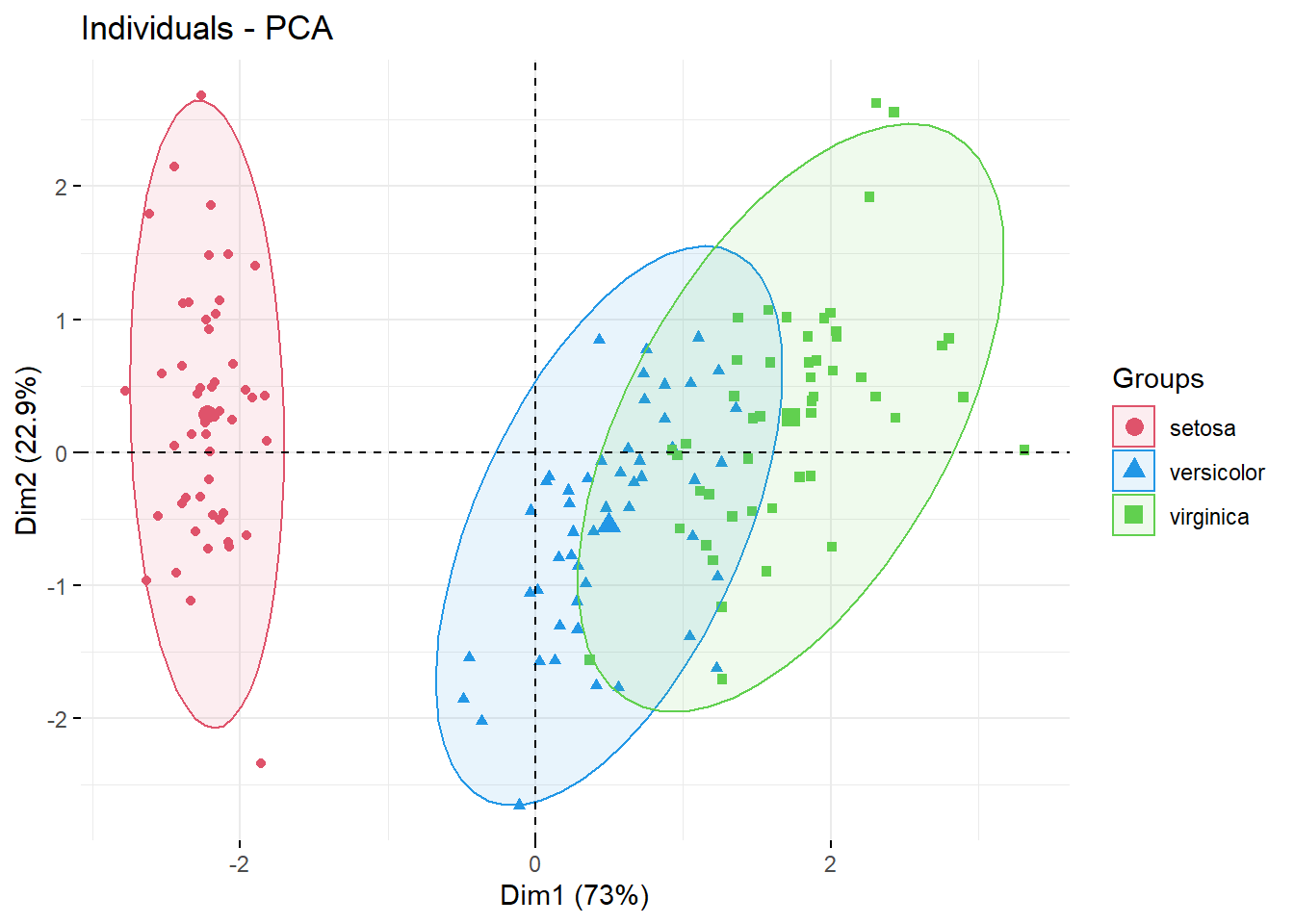However, some sets of points do not always follow elliptic shapes. Note, that FactoMineR represented the 2nd principal component upside-down compared to the very first PCA figure. To facilitate comparisons, the function plotPCAw has an argument allowing to rotate/flip any principal component axis. With more crowded data-sets it may be useful to rather highlight the more dense regions. For this reason this package proposes to use bagplots to highlight the region with 50% of data-points (in analogy to boxplots), a simple line draws the contour of the most distant points. ## via wrGraph, similar to FactoMineR but with bagplots plotPCAw(t(as.matrix(iris[,-5])), gl(3,50,labels=c("setosa","versicolor","virginica")), tit="Iris data", rowTyName="types of leaves", suplFig=FALSE, cexTxt=1.3, rotatePC=2)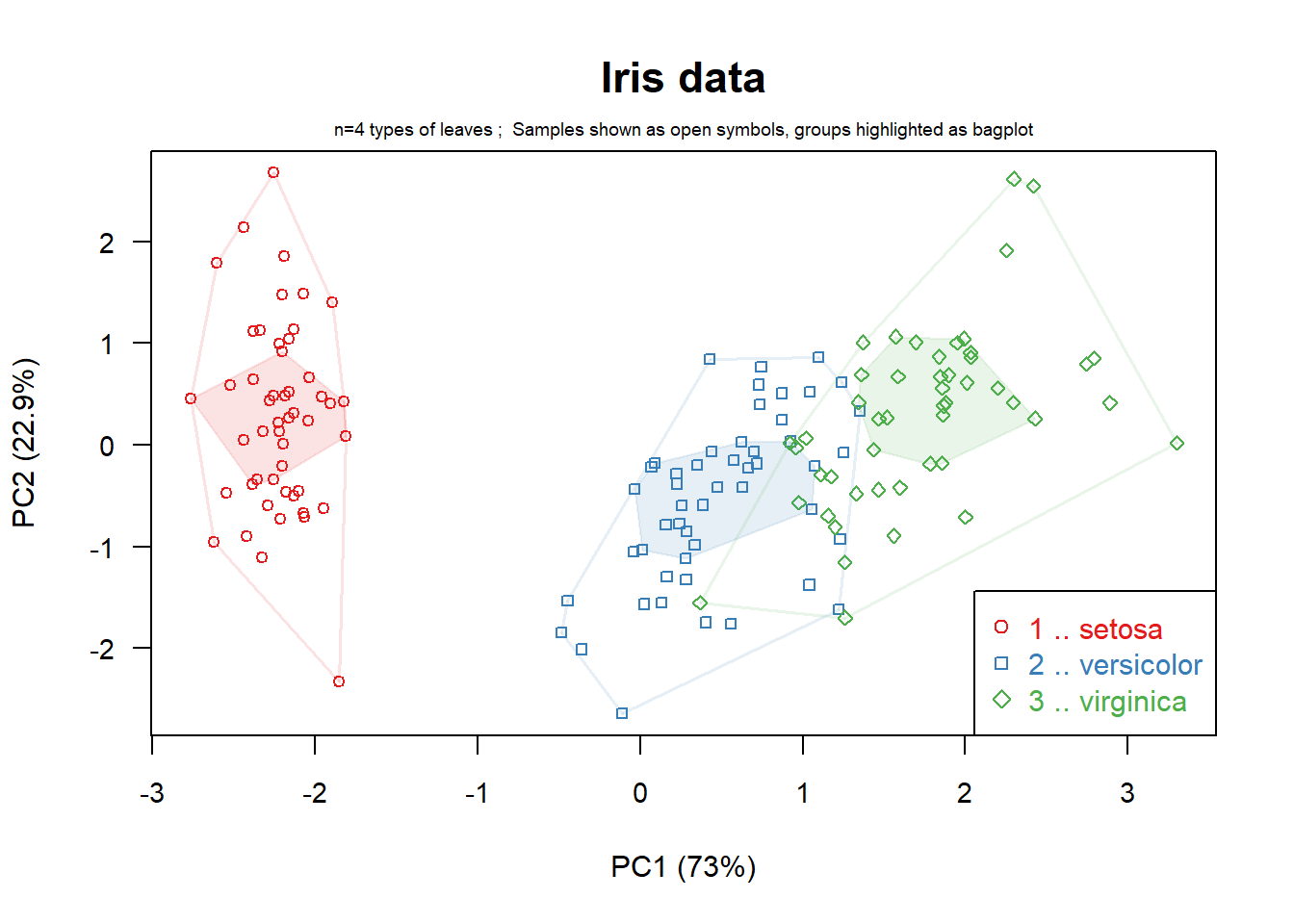Thus, you can see in this case, there is some intersection between versicolor and virginica species, but the center regions stay apart. Of course, similar to boxplots, this representation is nor adopted/recommended for multi-modal distributions within one group of points. One might get some indications about this by starting the data-analysis by inspecting histograms or vioplots for each set/column of data. You can also add the 3rd principal component and the Scree-plot : ## including 3rd component and Screeplot plotPCAw(t(as.matrix(iris[,-5])), gl(3,50,labels=c("setosa","versicolor","virginica")), tit="Iris data", rowTyName="types of leaves", cexTxt=2)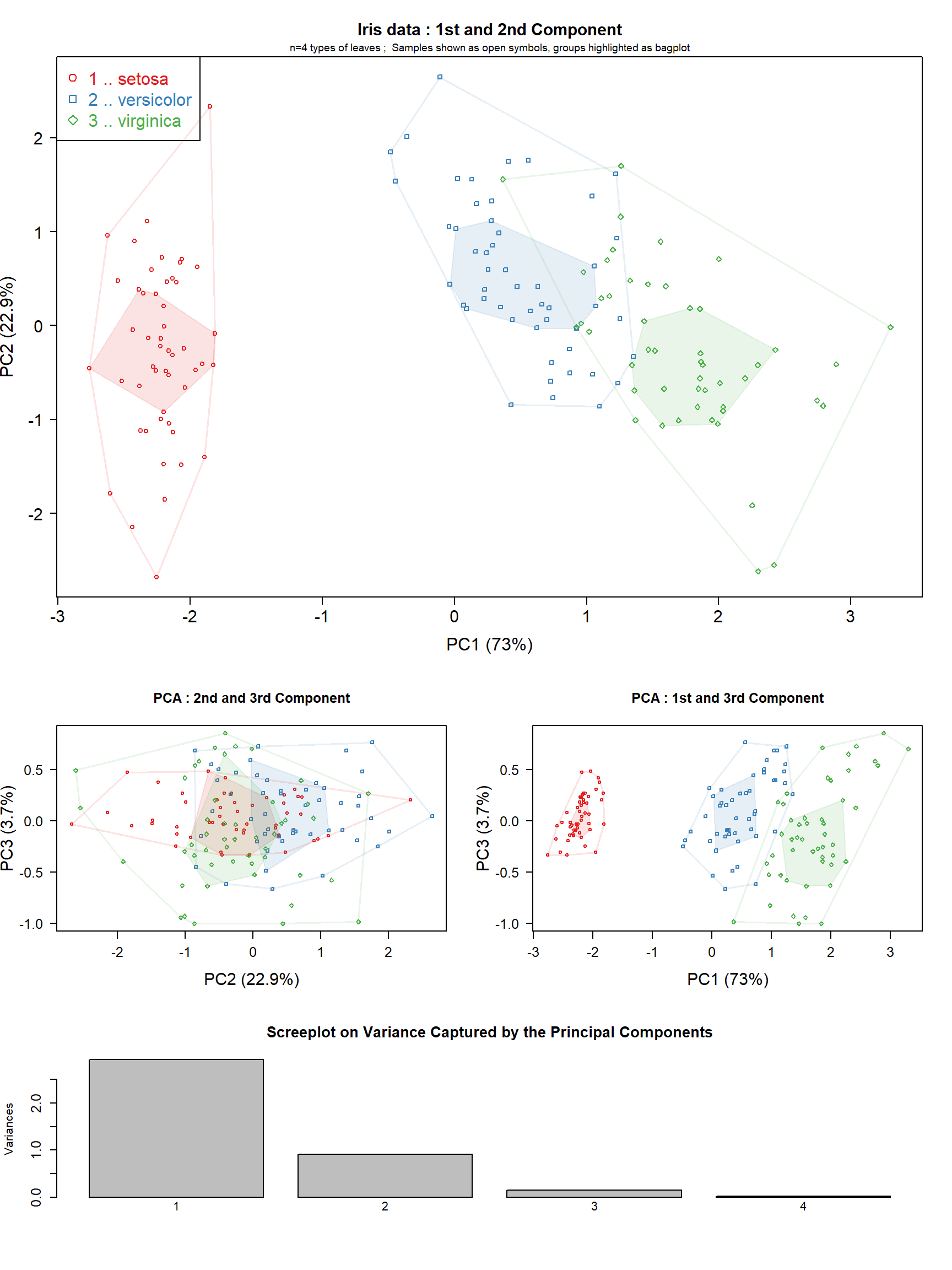## MA-Plot The aim of MA-plots consists in displaying a relative change on one axis (ordinate) while showing the absolute (mean) value on the x-axis (abscissa). This plot is very useful when inspecting larger data-sets for random or systematic effects, numerous implementations for specific applications exist (eg plotMA in DEseq2). The version presented here is rather generic and uses transparent points to avoid getting plots too crowded. First, let’s generate some toy data: ## toy data set.seed(2005); mat <- matrix(round(runif(2400),3), ncol=6) mat[11:90,4:6] <- mat[11:90,4:6] +round(abs(rnorm(80)),3) mat[11:90,] <- mat[11:90,] +0.3 dimnames(mat) <- list(paste("li",1:nrow(mat),sep="_"),paste(rep(letters[1:2],each=3),1:6,sep="")) ## assume 2 groups with 3 samples each matMeans <- round(cbind(A=rowMeans(mat[,1:3]), B=rowMeans(mat[,4:6])),4) One way of using the function MAplotW() is by providing (explicitely) the M- and A-values. By default a threshold-line is drawn for a fold-change of 1.5x (which on log2 scale apprears at +/- 0.58). ## now we are ready to plot, M-values can be obtained by subtracting thr group-means MAplotW(M=matMeans[,2] -matMeans[,1], A=rowMeans(mat))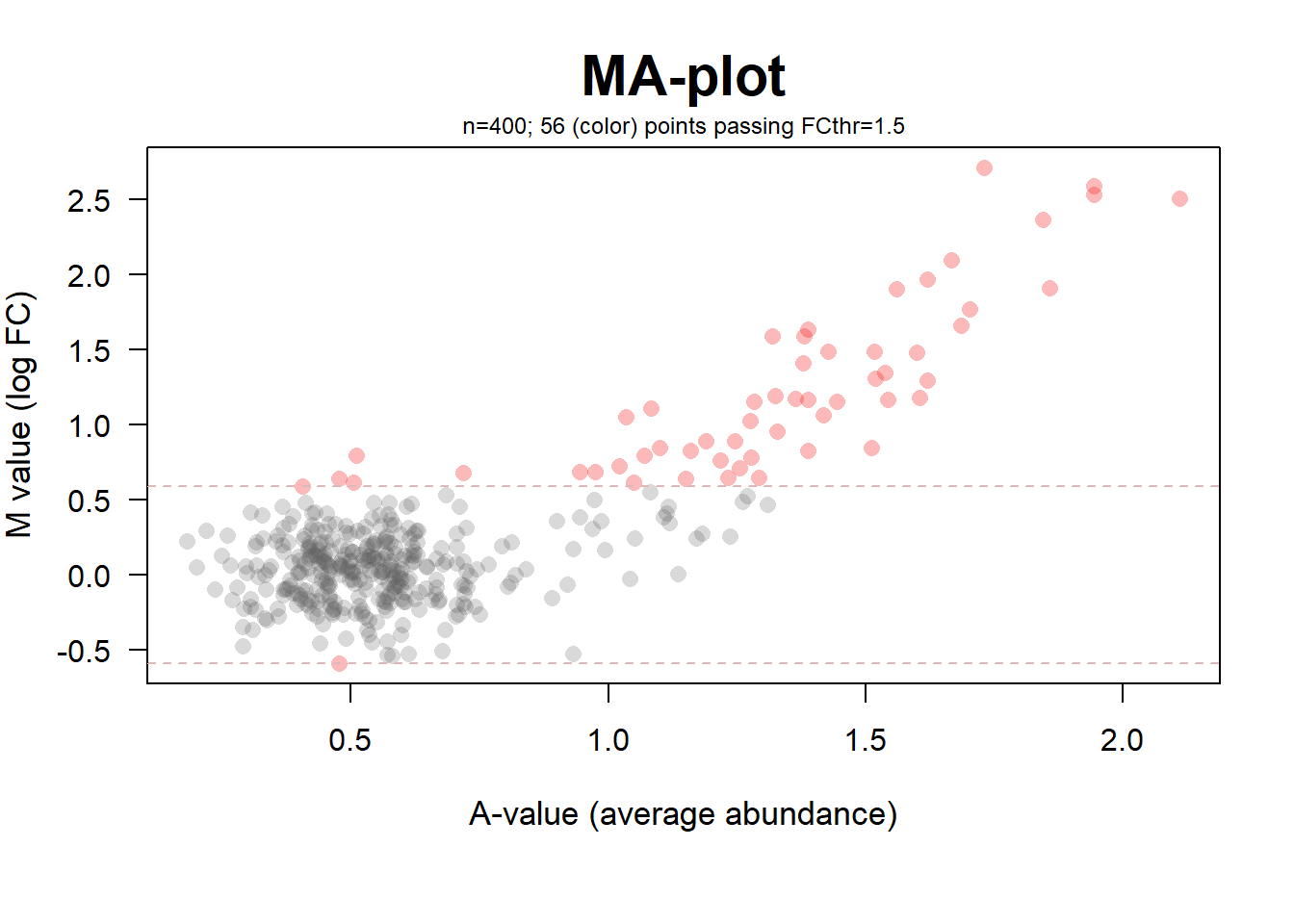The function MAplotW may also be used to conveniently inspect results from t-tests performed with the package wrMisc or from the very popular package limma. ## assume 2 groups with 3 samples each and run moderated t-test (from package 'limma') tRes <- wrMisc::moderTest2grp(mat, gl(2,3), addResults=c("FDR","Mval","means")) This object conatains data for different types of plots (MA-plot, Volcano-Plot, etc ..), let’s also mark the names of those passing the fold-change threshold. ## convenient way, change fold-change threshold to 2x and mark who is beyond : MAplotW(tRes, FCth=2, namesNBest="passFC") #> -> MAplotW : Successfully extracted 400 Mvalues and 400 Avalues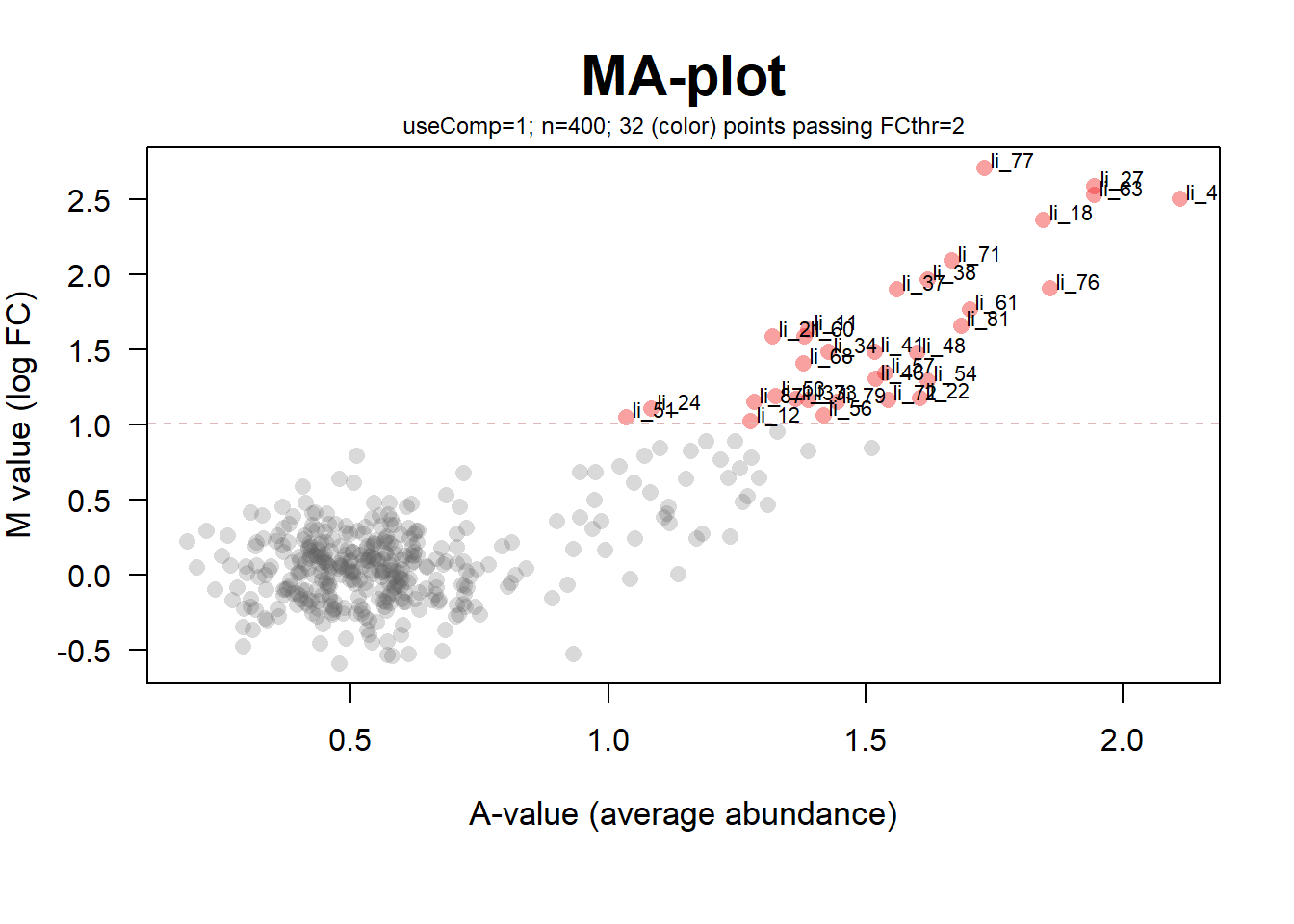In the plot above one can see easily most of the points from the second group which have been increased in lines 11 to 90, many of them exceed a (based on linear data) fold-change of 2 (which on log2 scale appears at 1.0). ## Volcano-Plot The aim of Volcano-plots is to display a log-ratios on the x-axis (ordinate) and outcome of statistical test on the other axis (abscissa). Typically the statistical values are represented as negative log10. This plot is very useful when inspecting larger data-sets for random or systematic effects. Points with high log-ratios (ie high fold-change) may not always have enthusiastic p-values, too. Many times such divergences may point to high intra-group variability. Numerous implementations for specific applications exist (eg the package EnhancedVolcano). The version presented here is rather generic and uses transparent points to avoid getting plots too crowded. Furthermore, this version has the advantage to take all information needed directly in MArrayLM-objects, like the output of moderTest2grp() or moderTestXgrp(). ## let's generate some toy data library(wrMisc) set.seed(2005); mat <- matrix(round(runif(900),2), ncol=9) rownames(mat) <- paste0(rep(letters[1:25],each=4), rep(letters[2:26],4)) mat[1:50,4:6] <- mat[1:50,4:6] + rep(c(-1,1)*0.1,25) mat[3:7,4:9] <- mat[3:7,4:9] + 0.7 mat[11:15,1:6] <- mat[11:15,1:6] - 0.7 ## assume 2 groups with 3 samples each gr3 <- gl(3,3,labels=c("C","A","B")) tRes2 <- moderTest2grp(mat[,1:6], gl(2,3)) #> Warning in fdrtool::fdrtool(z, statistic = "pvalue", plot = FALSE, verbose #> = !silent): There may be too few input test statistics for reliable FDR #> calculations! #> Warning: Censored sample for null model estimation has only size 1 ! #> Warning in fdrtool::fdrtool(z, statistic = "pvalue", plot = FALSE, verbose #> = !silent): There may be too few input test statistics for reliable FDR #> calculations! VolcanoPlotW(tRes2) #> -> VolcanoPlotW : Using element 'FDR' as FDR-values for plot #> -> VolcanoPlotW : Successfully extracted 100 Mvalues and 100 pValues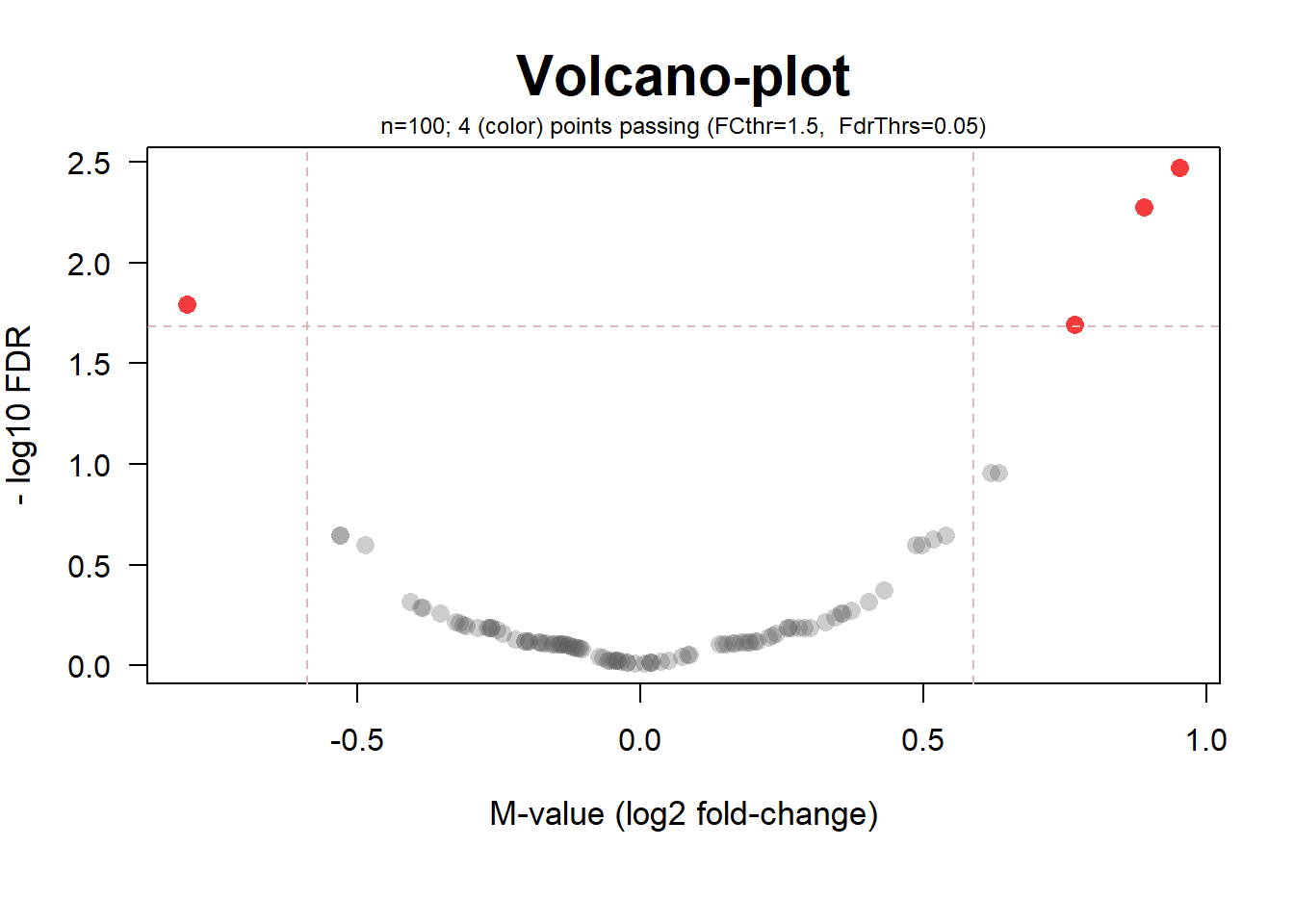VolcanoPlotW(tRes2, FCth=1.3, FdrThrs=0.2, namesNBest="pass") #> -> VolcanoPlotW : Using element 'FDR' as FDR-values for plot #> -> VolcanoPlotW : Successfully extracted 100 Mvalues and 100 pValues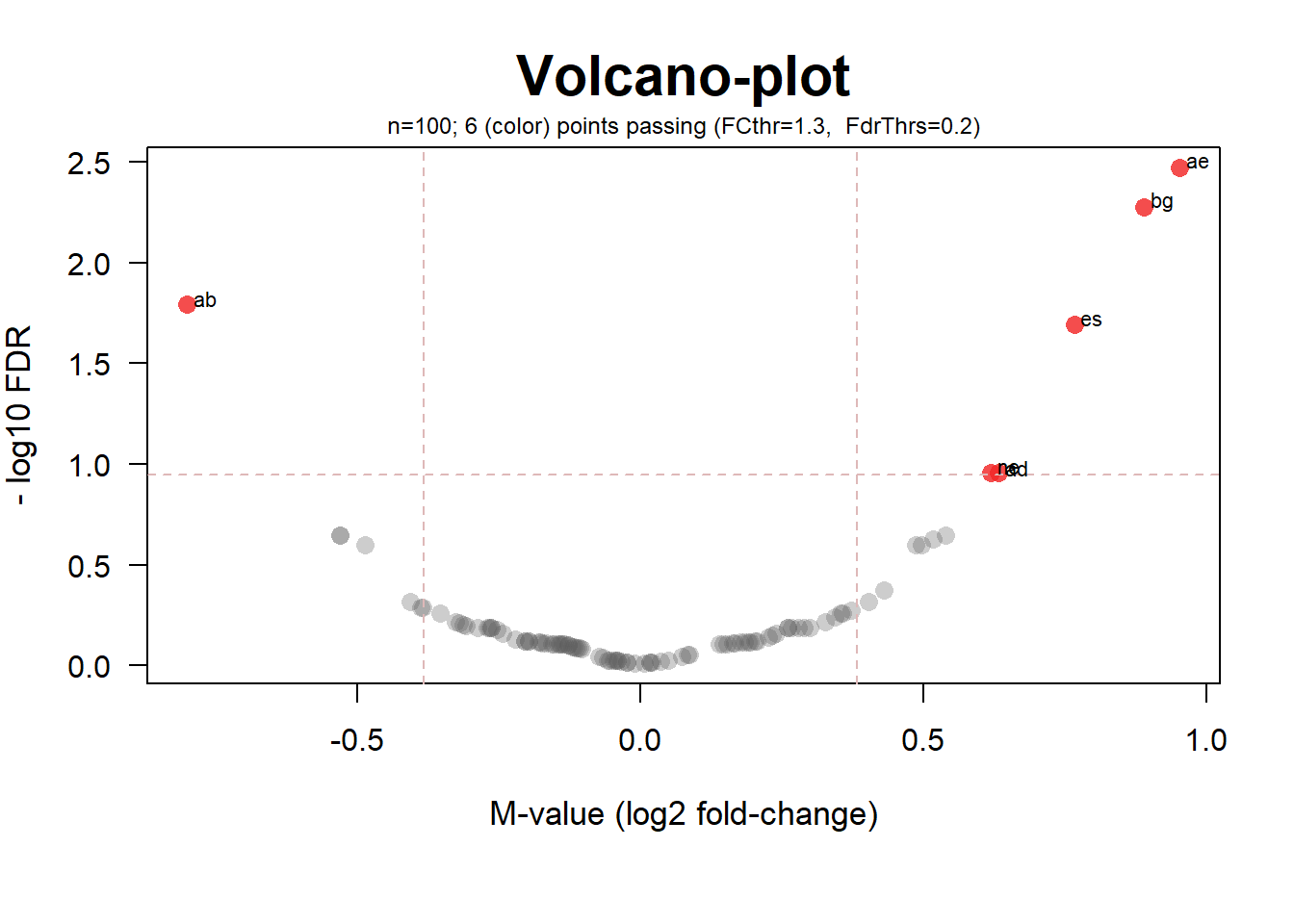Note, that this exampe is very small and for this reason the function fdrtool() used internally issues some warnings since the estimation of lfdr is not optimal. ## assume 3 groups with 3 samples each tRes <- moderTestXgrp(mat, gr3) #> Warning in fdrtool::fdrtool(z, statistic = "pvalue", plot = FALSE, verbose #> = !silent): There may be too few input test statistics for reliable FDR #> calculations! #> Warning in fdrtool::fdrtool(z, statistic = "pvalue", plot = FALSE, verbose #> = !silent): There may be too few input test statistics for reliable FDR #> calculations! #> Warning in fdrtool::fdrtool(z, statistic = "pvalue", plot = FALSE, verbose #> = !silent): There may be too few input test statistics for reliable FDR #> calculations! layout(matrix(1:2, nrow=1)) VolcanoPlotW(tRes, FCth=1.3, FdrThrs=0.2, useComp=2) #> -> VolcanoPlotW : Using element 'FDR' as FDR-values for plot #> -> VolcanoPlotW : Successfully extracted 100 Mvalues and 100 pValues VolcanoPlotW(tRes, FCth=1.3, FdrThrs=0.2, useComp=3) #> -> VolcanoPlotW : Using element 'FDR' as FDR-values for plot #> -> VolcanoPlotW : Successfully extracted 100 Mvalues and 100 pValues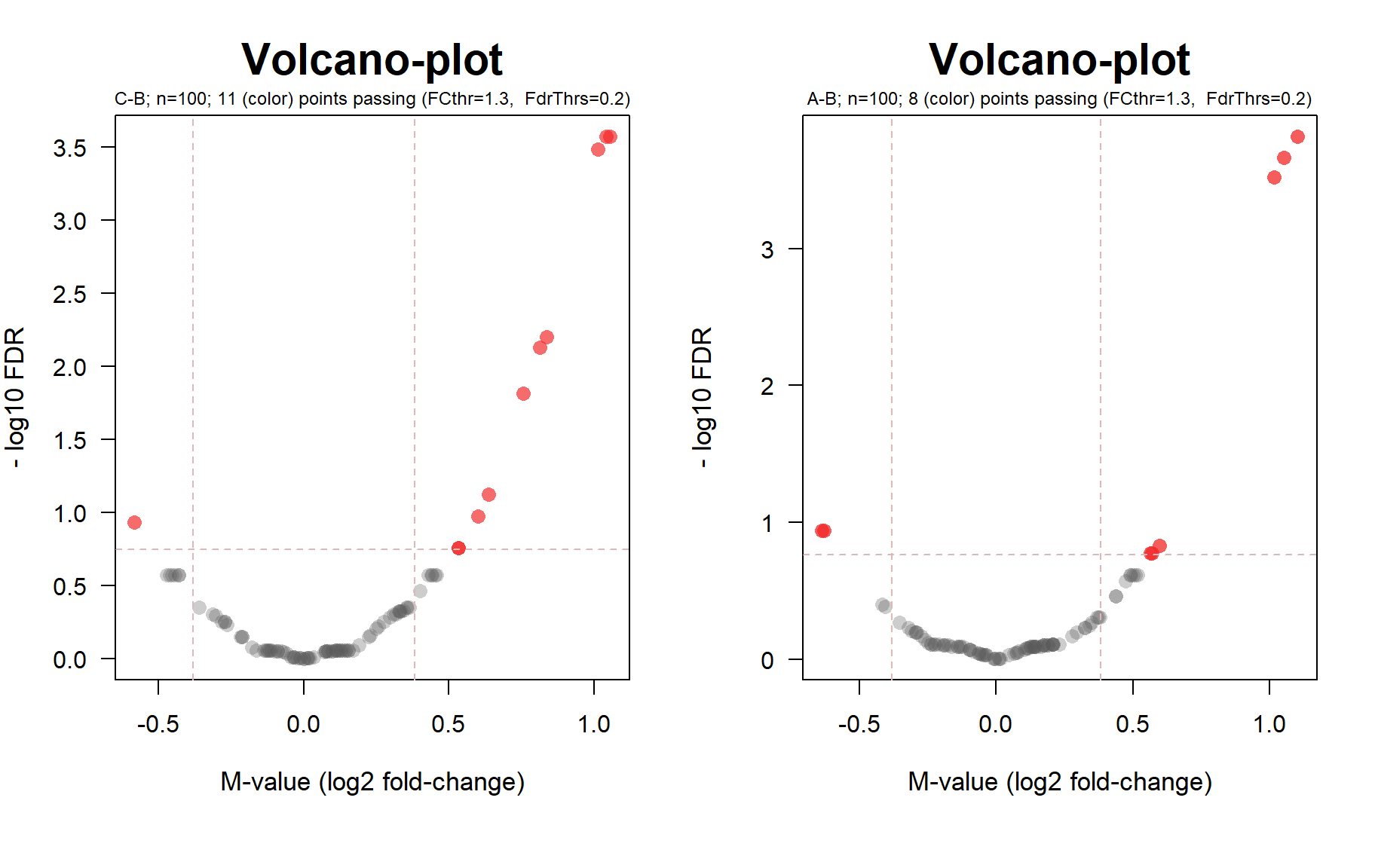## Standalone html Page with Plot and Mouse-Over Interactive Features The idea of this function dates before somehow similar applications were made possible using Shiny. In this case the original idea was simply to provide help to identify points in a plot and display via mouse-over labels to display and by providing clickable links. To do so, very simple html documents are created which display a separately saved image and have a section indicating at which location of the figure which information should be displayed as mouse-over and providing the clickable links. In high-throughput biology many times researchers want quickly know which protein or gene a given point in a graphic corresponds and being able to get further information on this protein or gene via links to UniProt or GenBank. As this functionality was made for separate html documents it’s output cannot be easily integrated into a vignette like this one. To generate such a simple mouse-over interactive html document, the user is invited to run the code of this vignette. At the last step you could ask R to open the interactive html page in the default browser. Concerning the path provided in the argument pngFileNa of the function mouseOverHtmlFile: In a real world case, you might want to choose other locations to save files rather than R-temp which will be deleted when closing your instance of R. It is also possible to work with relative paths (eg by giving the png-filename without path). Then the resultant html will simply require the png-file to be in the same directory as the html itself, and similarly for the clickable links. This results in files that are very easy to distribute to other people, in particular if the clickable links are pointing to the internet, however, the pnh-file always needs to be in the same directory as the html … If you have the possibility to make the png-file accessible through a url, you could also provide this url. The example below shows usage when specifying absolute paths. Please note that the resulting html will not display the image if your browser cannot access the image any more. ## Let's make some toy data df1 <- data.frame(id=letters[1:10], x=1:10, y=rep(5,10) ,mou=paste("point",letters[1:10]), link=file.path(tempdir(),paste(LETTERS[1:10],".html",sep="")),stringsAsFactors=FALSE) ## here we'll use R's tempdir, later you may want to choose other locations pngFile <- file.path(tempdir(),"test01.png") png(pngFile, width=800, height=600, res=72) ## here we'll just plot a set of horiontal points ... plot(df1[,2:3], las=1, main="test01") dev.off() #> png #> 2 ## Note : Special characters should be converted for proper display in html during mouse-over library(wrMisc) df1$mou <- htmlSpecCharConv(df1$mou) ## Let's add the x- and y-coordiates of the points in pixels to the data.frame df1 <- cbind(df1, convertPlotCoordPix(x=df1[,2], y=df1[,3], plotD=c(800,600), plotRes=72)) #> -> convertPlotCoordPix : supl params : mar=6.2,4,4,2 dim=800x600 res=72 head(df1) #> id x y mou link xPix #> 1 a 1 5 point a C:\\Users\\wraff\\AppData\\Local\\Temp\\RtmpGMhX3s/A.html 81 #> 2 b 2 5 point b C:\\Users\\wraff\\AppData\\Local\\Temp\\RtmpGMhX3s/B.html 155 #> 3 c 3 5 point c C:\\Users\\wraff\\AppData\\Local\\Temp\\RtmpGMhX3s/C.html 229 #> 4 d 4 5 point d C:\\Users\\wraff\\AppData\\Local\\Temp\\RtmpGMhX3s/D.html 303 #> 5 e 5 5 point e C:\\Users\\wraff\\AppData\\Local\\Temp\\RtmpGMhX3s/E.html 377 #> 6 f 6 5 point f C:\\Users\\wraff\\AppData\\Local\\Temp\\RtmpGMhX3s/F.html 451 #> yPix #> 1 284 #> 2 284 #> 3 284 #> 4 284 #> 5 284 #> 6 284 ## Now make the html-page allowing to display mouse-over to the png made before htmFile <- file.path(tempdir(),"test01.html") mouseOverHtmlFile(df1, pngFile, HtmFileNa=htmFile, pxDiam=15, textAtStart="Points in the figure are interactive to mouse-over ...", textAtEnd="and/or may contain links") ## We still need to make some toy links for(i in 1:nrow(df1)) cat(paste("point no ",i," : ",df1[i,1]," x=",df1[i,2]," y=", df1[i,3],sep=""), file=df1$link[i])
## Now we are ready to open the html file using any browser ..
#from within R# browseURL(htmFile) 

## Acknowledgements

The author wants to acknowledge the support by the IGBMC (CNRS UMR 7104, Inserm U 1258, UdS), CNRS, Université de Strasbourg and Inserm and of course all collegues from the IGBMC proteomics platform. The author wishes to thank the CRAN-staff for all their help with new entries and their efforts in maintaining this repository of R-packages.

Thank you for you interest in this package. This package is constantly evolving, new functions may get added to the next version.

## Appendix: Session-Info

#> R version 4.1.0 (2021-05-18)
#> Platform: x86_64-w64-mingw32/x64 (64-bit)
#> Running under: Windows 10 x64 (build 19042)
#>
#> Matrix products: default
#>
#> locale:
#>  LC_COLLATE=C                   LC_CTYPE=French_France.1252
#>  LC_MONETARY=French_France.1252 LC_NUMERIC=C
#>  LC_TIME=French_France.1252
#>
#> attached base packages:
#>  stats     graphics  grDevices utils     datasets  methods   base
#>
#> other attached packages:
#>  dplyr_1.0.7      factoextra_1.0.7 ggplot2_3.3.5    FactoMineR_2.4
#>  wrGraph_1.2.5    wrMisc_1.6.0.2
#>
#> loaded via a namespace (and not attached):
#>   sass_0.4.0           tidyr_1.1.3          jsonlite_1.7.2
#>   carData_3.0-4        bslib_0.2.5.1        sm_2.2-5.6
#>   assertthat_0.2.1     highr_0.9            cellranger_1.1.0
#>  yaml_2.2.1           ggrepel_0.9.1        pillar_1.6.1
#>  backports_1.2.1      lattice_0.20-44      limma_3.48.1
#>  glue_1.4.2           digest_0.6.27        RColorBrewer_1.1-2
#>  ggsignif_0.6.2       colorspace_2.0-2     htmltools_0.5.1.1
#>  pkgconfig_2.0.3      broom_0.7.8          haven_2.4.1
#>  purrr_0.3.4          scales_1.1.1         openxlsx_4.2.4
#>  rio_0.5.27           fdrtool_1.2.16       tibble_3.1.2
#>  generics_0.1.0       farver_2.1.0         car_3.0-11
#>  ellipsis_0.3.2       ggpubr_0.4.0         DT_0.18
#>  withr_2.4.2          magrittr_2.0.1       crayon_1.4.1
#>  tidyselect_1.1.1     xfun_0.24